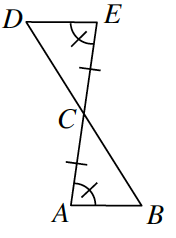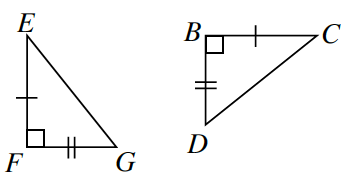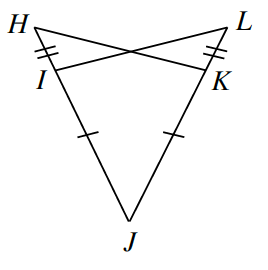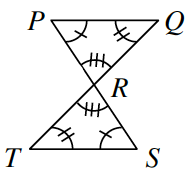### Home > CCG > Chapter Ch7 > Lesson 7.3.1 > Problem7-122

7-122.

For each pair of triangles below, determine if the triangles are congruent. If the triangles are congruent, state the triangle congruence condition that justifies your conclusion. If you cannot conclude that the triangles are congruent, explain why not.

1. $ΔCAB ≅ Δ\underline{\qquad}$$ΔCED$; vertical angles are equal, $\text{ASA}≅$.

1. $ΔCBD ≅ Δ\underline{\qquad}$$ΔEFG$; $\text{AAS}≅$

1. $ΔLJI ≅ Δ\underline{\qquad}$1. $ΔPRQ ≅ Δ\underline{\qquad}$